# Experimental study on thermal properties of bio-composite (gypsum plaster reinforced with palm tree fibers) for building insulation

Experimental study on thermal properties of bio-composite (gypsum plaster reinforced with palm tree fibers) for building insulation

Ines Amara*Atef Mazioud Ines Boulaoued Abdallah Mhimid

Thermal System Energetic Laboratory Research (LESTE), University of Monastir, National School Engineering of Monastir, Avenue Ibn Jazzar 5019, Tunisia

CERTES EA3481, University Paris Est Créteil, 61 av.du Général de Gaulle, Créteil 94010, France

Corresponding Author Email:
ines.amara@live.fr
Page:
576-584
|
DOI:
https://doi.org/10.18280/ijht.350314
|
Accepted:
|
Published:
30 September 2017
| Citation

OPEN ACCESS

Abstract:

This paper presents an experimental and theoretical study of the thermo physical properties of date palm tree fiber-reinforced plaster composites. In this work, the thermal conductivity and diffusivity of composites are determined by the “DICO” method. The palm tree fibers are incorporated, without any preliminary treatment, into a plaster mixture. Four samples containing different percentages of palm tree fibers are realized (prepared) and examined. Furthermore, the interface of palm tree fibers is observed by Scanning Electron Microscopy (SEM). The experimental results show that the reinforcement of plaster by palm fibers decreases the thermal conductivity and diffusivity. Therefore, the measured thermal conductivity is compared with the theoretical models (serial model, parallel model, Krischer model and Willy and Southwick model).

Keywords:

palm-tree-fiber, thermal conductivity, thermal diffusivity, dico method, modeling and measurement

1. Introduction

Due to the increasing population energy consumption in the sector of building and the hard economic situation, it is necessary to control energy during the building process. One of the many difficulties confronting researchers in the field of building is the uncovering methods that reduce energy consumption as well as the new and innovative techniques that decrease heat loss and enhance product quality.

An analysis of conductive heat transfer through the material is very important in an energy-efficient building design. Thus, the knowledge of thermal properties, particularly thermal conductivity and thermal diffusivity of material construction, are of great importance.

This paper studies the thermophysical properties of composite materials of plaster that incorporates palm tree fibers. Indeed, the latter are a natural and renewable product with good thermophysical and acoustic properties for the development of insulating materials. They come from the Mediterranean area (Tunisia, Algeria, Morocco, etc.).  A number of studies have shown that vegetable-fiber reinforced plaster materials possess good thermal properties. Toppi and Mozzarella  studied the thermal properties of gypsum-based composite materials with a micro-encapsulated phase change material. Mustapha Boumhaout et al.  studied the thermomechanical properties of a composite material based on mortar with a mesh of date palm fibers. A. Djoudi et al.  used a new composite material of construction made of plaster, sand, crushed gravel and date palm trees. The authors also proved a decrease in thermal conductivity and density. The same behavior was noticed by Abou Bakr Cherki  who studied the effect of adding cork on the thermal conductivity, thermal diffusivity and heat capacity of a composite material based on granular cork embedded in plaster. Their results show the interest of working with cork to reduce thermal conductivity and make a material that has a low heat transmission, compared to the plaster without cork. Another study was made by Soumia Mounir et al. , who characterized experimentally the behavior of thermal inertia and thermal properties for walls coated by sealants: white cement-cork, cement mortar-cork and plaster-cork. H. Lakrafli et al.  studied the effect of adding leather wastes, namely chrome shavings and buffing dust on the thermal properties of specimens cement and plaster composites. Their results indicated a significant decrease in density and thermal conductivity with an increase in content tannery wastes.

Several methods of steady measurements have been opted for in order to determine the thermal properties of porous materials through the use of the periodic method, the hot plate method, as well as other ones.  Rahmanain and Wang determined the thermal conductivity for gypsum at a high temperature, treating it as a porous material consisting of a solid material and pores using the hot plate method . Boulaoued et al.  used the box method to characterize the thermophysical properties of a sample prepared by mixtures of cement-date palm fibers. Lachheb et al.  utilized the periodic method to determine the thermal characterization of phase-change composite materials. Amel Limam et al.  determined the thermal properties of composite materials (Aleppo Pine wood, cork) using a heat flow meter apparatus.

In this work, the enhancement of the thermophysical properties of date-palm-tree-fiber reinforced plaster matrix is investigated. The thermal conductivity and diffusivity of the latter composite are measured by the DICO method. The effect of the addition of date palm fibers on the thermal conductivity and diffusivity of the plaster matrix is studied. Hence, the theoretical values of thermal conductivity obtained from the serial, parallel, Krischer, and Willy and Southwick models and those determined experimentally are compared.

2. Description of Used Materials

This work studies the thermophysical properties of a composite material made with gypsum and fibers of palm tree trunks. This reinforcement can offer a good thermal performance.

2.1 Gypsum

Plaster is a building material used for fire resistant walls and ceilings. Plaster starts as a dry powder similar to mortar or cement, and like those materials it is mixed with water to form a good paste.

These characteristics make plaster suitable for a finishing material, rather than a load-bearing material. The term “plaster” refers to gypsum plaster or hemi hydrated gypsum. Gypsum plaster is produced by heating gypsum to about 150°C as follows:

$2 \mathrm{CaSO}_{2} \cdot 4 \mathrm{H}_{2} \mathrm{O}+\mathrm{Heat} \rightarrow 2 \mathrm{CaSO}_{4} \mathrm{H}_{2} \mathrm{O}+3 \mathrm{H}_{2} \mathrm{O}$

The water-gypsum ratio (w/g) in the mixing process can vary from 0.6 to 0.8 or higher. When the dry plaster powder is mixed with water, it re-forms into gypsum. The gypsum used in this work is located in the vicinity of Tataouine city, Tunisia. It is mixed with water, using a water-gypsum plaster of 0.8 by mass. It has been found that this gypsum has a density of 988.240 kg/m3 corresponding to a thermal conductivity of 0.431 W/m K.

2.2 Date palm fibers (Trunk)

The natural fibers used in this research are the date palms collected in the oases of the Tunisian South. Palm tree fibers are natural and renewable with very interesting thermal properties thanks to their porosity. The part used in this work is the trunk, presented in figure 1. The fibers are separated into individual fibers of a diameter of 0.7-1.1 mm.

The fibers of palm trees are characterized by their chemical composition, which enormously influences their durability. The use of natural original vegetable fibers in construction is not new in its principle. Plaster was the first composite strengthened with vegetable fibers, then came cement and concrete.

The trunk of palm trees is constituted by three major components : cellulose, hemicellulose and lignin. The remainder of the composition comprises the extractable, water soluble and ash (mineral matter).

The properties of the reinforcements of used palm tree fibers are summarized in Table 1.

## 1.png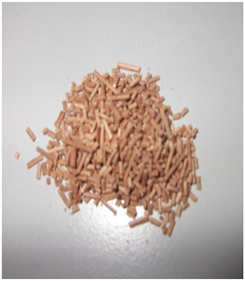Figure 1. Fibers after being pulled out from date-palm trunks

Table 1. Characteristics of used palm fibers

 Reinforcement Date palm fibers Type Trunk Density 289.588 kg/m3 Thermal conductivity 0.17 W/m K
3. Preparation of Samples

The objective of this work is to improve the thermal properties of gypsum, which may be used in walls, so four samples are prepared corresponding to four different date palm wood percentages (2%, 5%, 8% and 10%), to consider the date palm content dependence on the thermal properties of composite materials (Figure 2).

## 2.png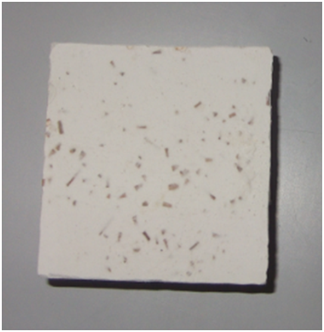Figure 2. Example of composite samples

Table 2. Dry density of five studied composites

 Palm mass fibers (%) Dry density (kg/m3) 0 988.240 2 976.098 5 894.735 8 857.132 10 825.233

Furthermore, a sample of gypsum plaster without palm tree fibers is prepared, having 44 × 44 × 12 mm3 dimensions, in order to compare the variation in the thermal properties of palm-tree-fiber-gypsum with those of gypsum without palm tree fibers. For all samples, the percentages for water used in

the plaster is (W/g = 0.8). The dimensions and density of all studied samples are summarized in Table 2.

4. Description of Method Used for Thermal Characterization: Dico

The measurements of thermophysical properties, like thermal conductivity and thermal diffusivity, are obtained using the periodic method DICO, detailed in the literature . The general principle of the experimental set-up is illustrated in figure 3.

The method is based on the use of a low temperature modulation in a parallelepiped-shaped sample (44mm × 44mm × 12mm) and allows obtaining all these thermo physical parameters in only one measurement with their corresponding statistical confidence bounds [12-13].

## 3.pngFigure 3. Experimental set-up of periodic method: DICO

The composite sample is fixed between two metallic plates (brass to the lower plate and copper to the top plate) (figure 4). A good thermal exchange and contact between the different elements and the sample is ensured by using conducting grease. The front side of the first metallic plate is heated periodically using a sum of five sinusoidal signals. The temperature is measured using thermocouples placed inside both metallic plates . The thermophysical parameters of the composite material are identified by comparing between the experimental and theoretical heat transfer functions . The system under consideration is modeled by one dimensional quadruple theory . The experimental heat transfer function is calculated at each excitation frequency as a ratio between the Fourier transform temperature of the front and rear plates.

5. Effective Thermal Conductivity Models

Different types of theoretical models have been developed in the literature to predict the effective thermal conductivity of three-phase mixtures.

In this work, a composite material is taken according to the following hypotheses of simplification:

Convection is negligible inside the pores;

Temperature is low;

The material is opaque;

The physical characteristics are constant;

The mass diffusion is absent;

The term source is absent.

## 4.pngFigure 4. Schematic of experimental method

5.1 Effective thermal conductivity of composite according to series and parallel models

In this approach, it is considered that the material is formed by a solid phase constituted by plaster and fibers of palm trees, a gaseous phase (the pores contain air) and a liquid phase (water in general). Consequently, the concept of the effective thermal conductivity of a solid1 (plaster matrix) $\lambda_{S 1}$ , a solid2 (palm tree fibers) $\lambda_{s 2}$ , a liquid phase $\lambda_{l}$ and a gaseous phase $\lambda_{g}$ is introduced. The effective thermal conductivity of the serial model is given by:

$\lambda_{e f f s}=\frac{1}{\frac{\varepsilon_{s 1}}{\lambda_{s 1}}+\frac{\varepsilon_{s 2}}{\lambda_{s 2}}+\frac{\theta}{\lambda_{l}}+\frac{(\varepsilon-\theta)}{\lambda_{g}}}$        (1)

Moreover, the effective thermal conductivity of the parallel model is given by the following expression:

$\lambda_{e f p}=\varepsilon_{s 1} \lambda_{s 1}+\varepsilon_{s 2} \lambda_{s 2}+\theta \lambda_{l}+(\varepsilon-\theta) \lambda_{g}$     (2)

where $\mathcal{E}$ is the total porosity, $\varepsilon_{s 1}$ is the fraction of the solid1, $\varepsilon_{S} 2$ is the fraction of the solid2, and θ is the moisture content.

Introducing the mass ratio between the solid1 and the solid2 defined by:

$R_{m}=\frac{m_{s 2}}{m_{s 1}}$        (3)

The expressions of the fraction of the solid1 and solid2 are obtained as follows:

$\varepsilon_{s 1}=\frac{1-\varepsilon}{1+R_{m} \frac{\rho_{s 1}}{\rho_{s 2}}}$        (4)

$\varepsilon_{\mathrm{s} 2}=1-\varepsilon-\varepsilon_{\mathrm{s} 1}$      (5)

Finally, the effective thermal conductivity of the sample shown by the serial and parallel arrangement is given by:

$\lambda_{e f f s}=\frac{1}{\left(\frac{1-\varepsilon}{1+R_{m} \frac{\rho_{s 1}}{\rho_{s 2}}}\right) \frac{1}{\lambda_{s 1}}+\frac{1-\varepsilon-\varepsilon_{s 1}}{\lambda_{s 2}}+\frac{\theta}{\lambda_{l}}+\frac{(\varepsilon-\theta)}{\lambda_{g}}}$   (6)

and

$\lambda_{e f f p}=\left(\frac{1-\varepsilon}{1+R_{m} \frac{\rho_{s 1}}{\rho_{s 2}}}\right) \lambda_{s 1}+\left(1-\varepsilon-\frac{1-\varepsilon}{1+R_{m} \frac{\rho_{s 1}}{\rho_{s 2}}}\right)$ $\lambda_{s 2}+\theta \lambda_{7}+(\varepsilon-\theta) \lambda_{g}$      (7)

When the material is dry, we use the following expressions are used:

$\lambda_{e f f s}=\frac{1}{\left(\frac{1-\varepsilon}{1+R_{m} \frac{\rho_{s 1}}{\rho_{s 2}}}\right) \frac{1}{\lambda_{s 1}}+\frac{1-\varepsilon-\varepsilon_{s 1}}{\lambda_{s 2}}+\frac{\varepsilon}{\lambda_{g}}}$        (8)

$\lambda_{e f f p}=\left(\frac{1-\varepsilon}{1+R_{m} \frac{\rho_{s 1}}{\rho_{s 2}}}\right) \lambda_{s 1}+\left(1-\varepsilon-\frac{1-\varepsilon}{1+R_{m} \frac{\rho_{s 1}}{\rho_{s 2}}}\right) \lambda_{s 2}+\varepsilon \lambda_{g}$        (9)

## 5.png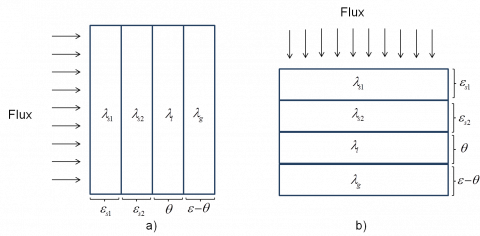Figure 5. a) Serial model; b) Parallel model

5.2 Effective thermal conductivity of composite according to mixed model

The effective thermal conductivity obtained for the parallel and serial models were used in other analytical models proposed by Krischer, and Willy and Southwick, which are described in the following paragraphs.

5.2.1 Krischer model

The effective thermal conductivity, according to the Krischer model , is a combination between the serial and parallel models (figure 7) by a blending factor, named the phase distribution factor ( $\mathcal{U}$ ). Researchers suppose that the volume fraction $\mathcal{U}$ of the layers oriented perpendicularly to the direction of the heat flow is arranged in series with the complementary fraction ( 1 - $\mathcal{U}$ ) of the layers oriented in parallel to the direction of the heat flow. The following equation describes the Krischer model:

$\lambda_{e f f k}=\frac{1}{\frac{u}{\lambda_{e f f p}}+\frac{(1-u)}{\lambda_{e f f s}}}$      (10)

Carson JK  and Harouna Bal et al.  utilized the Krischer model to predict the effective thermal conductivity in porous food and in laterite based bricks, respectively. The effective thermal conductivity predicted by the Krischer model was in good agreement with the experimental data.

## 6.png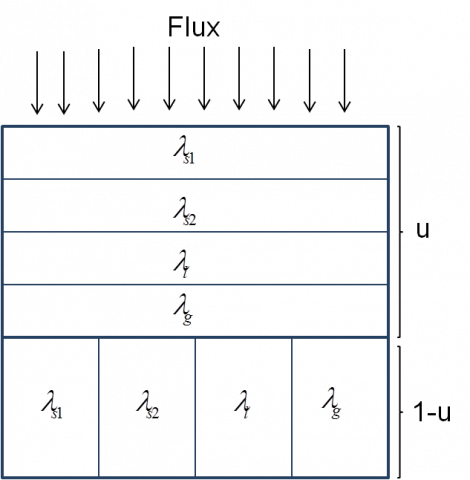Figure 6. Krischer model

## 7.png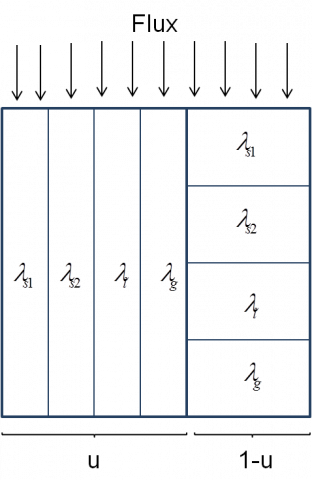Figure 7. Willy and Southwick model

Thus, when u=1, the Krischer model will be reduced to the series model; but when the parameter $\mathcal{U}$ is zero, the Krischer model will be reduced to the parallel model. Therefore, this model may be expected to provide some acceptable predictions of the thermal conductivity for the porous media. The value of $\mathcal{U}$ used in this study is u=0.25, which has been proposed by Amara et al. (2017) .

5.2.2 Willy and Southwick model

Willy and Southwick (1954)  put forward a model of perpendicular heat flux traversing parallel layers disposed in parallel with other layers in series:

$\lambda_{e f f W S}=u \lambda_{e f f p}+(1-u) \lambda_{e f f s}$       (11)

where u is assumed to be a numerical correlation factor and may be defined as a fraction of the material oriented in the direction of the heat flow, and the remaining (1-u) fraction is oriented perpendicular to the direction of the heat flow (Figure 8). The same value of u, suggested by , is used.

6. Results and Discussion

6.1 SEM analysis

The Scanning Electron Microscope (SEM) images of a trunk fiber are depicted in figure 8. This study enables knowing the form of the used fibers and having an idea of their dispersion in the produced mixtures. The microscope examinations are carried out using a JEOL JSM-6301F scanning electron microscope.

Observing these microstructures of date palm fibers, it can be seen that the surface of samples is irregular with many filaments, impurities, cells and pores, permitting the adhesion between the fiber and the plaster matrix.

Those observations are similar to those brought back in the literature on the fibers extracted from date palm trees [22, 23]. Indeed, all palm-trees fibers have a cylindrical form, and each fiber consists of multi cellular fibers containing a central void (lumen). Otherwise, the structure and the form of date palm trees are similar to those of coir fibers [23, 24].

## 8.png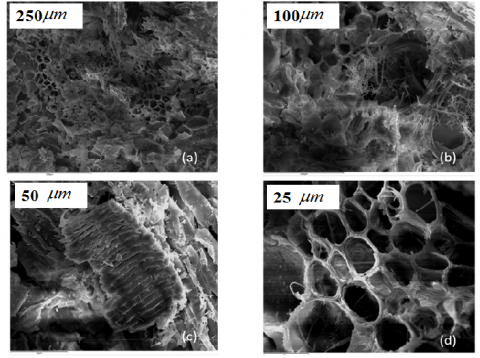Figure 8. SEM view of a trunk fiber. Scale bar equal to: (a) 250 $\mu m$, (b) 100 $\mu m$ , (c) 50 $\mu m$ , (d) 25 $\mu m$

It can be concluded that the holes confirm that the sample structure is very interesting for a good adhesion between date palm trees and some matrices. Thus, the date palm tree can be a good candidate for the development of suitable composites.

6.2 Thermophysical properties

In this study, the physical characteristics of different elements (air, water) are taken from the literature. Hence, the thermal conductivity of plaster and date palm trees is determined by the DICO method. As a result, the density of plaster is $\rho_{s 1}$ =988.240 kg/m and the thermal conductivity of plaster is $\lambda_{S 1}$=0.431 W/mK.

## 9.pngFigure 9. Comparison of experimental evolution of effective thermal conductivity as a function of fiber mass ratio with simulated Krischer model for varied porosity

The density and the thermal conductivity of date-palm-tree fibers are determined by the DICO method. Accordingly, the density and the thermal conductivity of fibers are respectively

$\rho_{s 2}=289.588 \mathrm{kg} / \mathrm{m}$ and $\lambda_{s 2}=0.17 \mathrm{W} / \mathrm{m} \mathrm{K}$ .

In the present work, the best porosity $\varepsilon=0.2$ (see figure 9) is used.

6.2.1 Thermal conductivity

Table 3 summarizes the experimental values of thermal conductivity and those calculated theoretically by the proposed models. It may be noted that plaster plays the role of a matrix and palm-trees fibers play the role of an insulator with $\lambda$ =0.17 W/mK. The effect of incorporating palm-tree fibers in plaster is to decrease its thermal conductivity.

The drop in thermal conductivity can be explained by the fact that the thermal conductivity of palm-tree-fibers is very low compared to that of plaster. Furthermore, the decline in density entrains a reduction in thermal conductivity. This dependence is always verified on materials with a plaster matrix and vegetable fibers according to Cherki et al.  who used granular cork bound with plaster, and according to Ashour et al.  who used matrix plaster reinforced by barley straws.

The comparison of the experimental measurements of the conductivity to theoretical approaches indicates that the theoretical results (serial, parallel, Krischer and Willy and Southwick model) correspond to the values obtained during the experiments. Moreover, it is found that the percentage deviation is less for the Krischer model. On this basis, the presented theoretical models can be used by engineers and researchers to predict the thermal conductivity of the composite at a given dosage of palm-tree fibers.

Table 3. Thermal conductivity of composite (plaster + palm tree fibers)

 Mass ratios of palm fibers (%) $\lambda_{e x p}$ (W/mK) Krischer model Willy and Southwick model $\lambda_{e f f K}$ (W/mK) Error (%) $\lambda_{e f f W S}$ (W/mK) Error (%) 0 0.431 0.431 0.00 0.431 0.00 2 0.304 0.224 26.3 0.144 52.6 5 0.252 0.216 14.3 0.139 44.8 8 0.237 0.209 11.8 0.135 43.0 10 0.232 0.205 11.6 0.132 43.1

## 10.png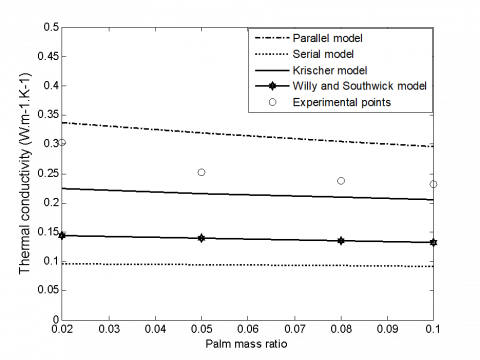Figure 10. Comparison between experimental evolution of thermal conductivity as a function of fiber mass ratio and thermal conductivity and theoretical models (serial, parallel, Krischer and Wily and Southwick models)

The most insulating material is observed with 10% by mass of date palm fibers. The thermal conductivity of composites goes down from 0.431 W/m K for the sample without fibers to 0.232 W/m K for the most insulating material. In addition, it has the advantage of being a natural, harmless and less-expensive product.

Figure 10 presents a comparison between the experimental evolution of the thermal conductivity as a function of the mass ratio of fibers and the one predicted by theoretical models (parallel, serial, Krischer and Willy and Southwick model).

As it can be seen, the Krischer model approaches better measurement results. The Krischer model considers both layers in parallel and serial layers, which seems more logical. This confirms the concordance between the theoretical and experimental values. It can be concluded that the values calculated by the Krischer model are relatively comparable to the experimental ones.

6.2.2 Thermal diffusivity

The thermal diffusivity of the composite is an important parameter in order to estimate their potential use in building . The characterization results of each sample from the experimental method are summarized in figure 11. The thermal diffusivity is calculated according to the DICO method.

## 11.pngFigure 11. Variation in thermal diffusivity of palm-tree-based material with the fiber mass ratio

The thermal diffusivity measures the rate of heat propagation through one material. For palm-tree-fiber-based insulators, it is found that an increase in the fiber mass ratio leads to a decrease in the effective thermal diffusivity of the composite. It is clear that the value of the thermal diffusivity of the composite depends on matrix porosity [26, 8]. Consequently, the more the medium contains date palm fibers, the less it allows heat transmission. Thus, depending on this important result in thermal insulation, an insulating material should not only have low conductivity but must also allow delaying heat transmission.

In addition to presenting the experimental measurements of thermal diffusivity, they are compared with a theoretical model.

The calculated thermal diffusivity $\alpha_{\text { theoretical }}$ of a composite is related to its thermal conductivity $\lambda_{e f f K}$ by the following equation:

$\alpha_{\text {theoretical}}=\frac{\lambda_{\text {eff} K}}{\rho \cdot \mathcal{C}_{p}}$       (12)

where ρ and $c_{p}$ are the density and the heat capacity, respectively.

The values of specific heat, used to calculate thermal diffusivity, are calculated by the following equation:

$c_{p c}=\frac{\sum_{i} m_{i} \cdot c_{p i}}{\sum_{i} m_{i}}$     (13)

Besides, the density is experimentally measured.

Table 4 gives the values of the dry thermal diffusivity calculated utilizing the theoretical equation. The results demonstrate that the thermal diffusivity falls with the incorporation of fibers. This result is in agreement with the results found for thermal conductivity

Table 4. Thermal diffusivity of plaster-palm-tree fibers

 Mass ratios of palm fibers (%) $\alpha_{\exp }\left(10^{-7} m^{2} / s\right)$ $\alpha_{\text {theoretical}}\left(10^{-7} \mathrm{m}^{2} / \mathrm{s}\right)$ error %) 0 4.225 4.225 0 2 3.923 3.608 8.0 5 3.869 3.642 5.8 8 3.614 3.583 0.9 10 3.417 3.598 5.3
7. Conclusion

In this paper, some thermophysical properties, such as thermal conductivity and thermal diffusivity of date-palm-tree fiber reinforcement gypsum have been estimated. The goal is to use the composite materials in the manufacture of thermal insulation for buildings. Thermophysical property measurements of composites have been obtained using the method of a periodic temperature slope. The results have shown that the incorporation of date palm tree fibers induces a decrease in both thermal conductivity and thermal diffusivity. This demonstrates that the thermal conductivity of the insulating plate realized in our laboratory seems to be more important than date palm tree fibers.

Therefore, four analytical models have been utilized to determine the thermal conductivity. The results suggest that the values of obtained various materials are acceptable compared with the values given by the DICO method.

The original insulating materials using palm tree fibers in a plaster matrix presents properties that are as a function of the fiber content mass ratio and have values that are generally comparable to synthetic insulating materials. It cannot be denied that the thermal conductivity and diffusivity of these insulators decline rapidly as a function of fiber content.

Acknowledgments

The authors would like to thank Mr. Patrick Ausset, the research engineer at the University of Paris-Est Créteil, France, for having made the palm tree fibers with the SEM observations.

Nomenclature
 Latin symbols cp specific heat capacity (J/kg K) m mass (kg) Rm mass ratio (%) u fraction of series arrangement (1-u) fraction of parallel arrangement Greek Symbols $\mathcal{E}$ porosity $\rho$ density (kg/m3) $\lambda$ thermal conductivity (W/m K) $\alpha$ thermal diffusivity (m2/s) Subscripts s1 Solid phase 1 s2 Solid phase 2 l Liquid phase g Gas phase eff effective effs Effective thermal conductivity of layers arranged in series effp Effective thermal conductivity of layers arranged in parallel effK Effective thermal conductivity of Krischer model effWS Effective thermal conductivity of Southwick model
References

 Toppi T., Mazzarella L. (2013). Gypsum based composite materials with microencapsulated PCM: Experimental correlations for thermal properties estimation on the basis of the composition, Energy Build, Vol. 57, pp. 227-236.

 Boumhaout M., Boukhattem L., Hamdi H., Benhamou B., Nouh F.A. (2017). Thermomechanical characterization of a bio-composite building material: Mortar reinforced with date palm fibers mesh, Construction and Building Materials, Vol. 135, pp. 241-250.

 Djoudi A., Khenfer M.M., Bali A., Kadri E.H., Debicki G. (2012). Performance of date palm fibers reinforced plaster concrete, International Journal of Physical Sciences, Vol. 7, No. 21, pp. 2845-2853.

 Cherki A.B., Khabbazi A., Remy B., Baillis D. (2013). Granular cork content dependence of thermal diffusivity, thermal conductivity and heat capacity of the composite material/Granular cork bound with plaster, Energy Procedia, Vol. 42, pp. 83-92.

 Mounir S., Abdelhamid K., Maaloufa Y. (2015). Thermal inertia for composite materials white cement-cork, cement mortar-cork, and plaster-cork, Energy Procedia, Vol. 74, pp. 991–999. DOI: 10.1016/j.egypro.2015.07.830

 Lakrafli H., Tahiri S., Albizane A., Otmani M.E.E. (2012). Effect of wet blue chrome shaving and buffing dust of leather industry on the thermal conductivity of cement and plaster based materials, Construction and Building Materials, Vol. 30, pp. 590–596. DOI: 10.1016/J.CONBUILDMAT.2011.12.041

 Rahmanian I., Wang Y.C. (2012). A combined experimental and numerical method for extracting temperature-dependent thermal conductivity of gypsum boards, Construction and Building Materials, Vol. 26, pp. 707-722. DOI: 10.1016/j.conbuildmat.2011.06.078

 Boulaoued I., Amara I., Mhimid A. (2016). Experimental determination of the thermo physical properties of new insulating Materials, International Journal of Heat and Technology, Vol. 3, No. 2, pp. 177-188.

 Lachheb M., Karkri M., Nasrallah S.B. (2015). Development and thermal characterization of an innovative gypsum-based composite incorporating phase change material as building energy storage system, Energy and Buildings, Vol. 107, No. 12, pp. 93-102.

 Amel L., Abdellatif Z., Daniel Q., Hebert S., Abdelkrim C. (2016). Experimental thermal characterization of bio-based materials (Aleppo Pine wood, cork and their composites) for building insulation, Energy and Buildings, Vol. 116, pp. 89-95.

 Sbiai A. (2011). Matériaux composites a matrice époxyde chargée par des fibres de palmier dattier: Effet de l’oxydation au tempo sur les fibres, Ph. D. dissertation, University of Lyon, France.

 Boudenne A., Ibos L., Géhin E., Candau Y. (2004). A simultaneous charactrisation of thermal conductivity and diffusivity of polymer materials by a periodic method, Journal of Physics D: Applied Physics, Vol. 37, No. 1, pp. 132-139.

 Lachheb M., Karkri M., Albouchi F., Mzaili F., Nasrallah S.B. (2014). Thermophysical properties estimation of paraffin/graphite composite phase change material using an inverse method, Energy Conversion & Management, Vol. 82, No. 6, pp. 229-237.

 Krupa I., Boudenne A., Ibos L. (2007). Thermophysical properties of polyethylene filled with metal coated polyamide particles, European Polymer Journal, Vol. 43, pp. 2443-2452. DOI: 10.1016/j.eurpolymj.2007.03.032

 Trigui A., Karkri M., Pen L., Boudaya C., Candau Y., et al. (2011). Thermal and mechanical properties of maize fibres–high density polyethylene biocomposites, Journal of Composite Materials, Vol. 47, No. 11, pp. 1387-1397.

 Maillet D., André S., Bastale J-C., Degiovanni A., Moyne C. (2000). Thermal Quadrupoles: Solving the heat equation through integral transforms, Wiley-Blackwell, p. 388.

 Krischer O, Kroll K. (1963). Technique du séchage. Berlin: Centre Technique des Industries Aérauliques et Thermiques (CETIAT). Traduction Springer-Verlag.

 Carson J.K. (2002). Prediction of the thermal conductivity in porous foods, Ph. D. dissertation, Food Engineering, Massey University, Palmerstone north, New Zealand.

 Bal H., Jannot Y., Gaye S., Demeurie F. (2013). Measurement and modelisation of the thermal conductivity of a wet composite porous medium: Laterite based bricks with millet waste additive, Construction and Building Materials, Vol. 41, pp. 586-593.

 Amara I., Boulaoued I., Mhimid A., Mazioud A. (2017). Study and modeling of effective thermal conductivity of building insulator materials, Special Topics & Reviews in Porous Media: An International Journal.

 Willy M.R.J., Southwick P.F. (1954). An experimental investigation of the SP and resistivity phenomena in dirty sands, J. Petrol. Technol., Vol. 6, No. 2, pp. 44-57.

 Al-Khanbashi A., Al-Kaabi K., Hammami A. (2005). Date palm fibers as polymeric matrix reinforcement: DPF/polyester composite properties, Polym Composite, Vol. 26, pp. 486–497.

 John M.J., Anandjiwala R.D. (2008). Recent developments in chemical modification and characterization of natural fiber reinforced composites, Polymer Composites, Vol. 29, No. 2, pp. 187-207.

 Rout J., Misra M., Tripathy S., Nayak S., Mohanty A. (2002). Surface modification of coir fibers. Ⅱ. Cu (Ⅱ)-IO-4 initiated graft copolymerization of acrylonitrile onto chemically modified coir fibers, Journal of Applied Polymer Science, Vol. 84, No. 1, pp. 75-82.

 Santos W.N.D. (2003). Effect of moisture and porosity on the thermal properties of a conventional refractory concrete, Journal of the European Ceramic Society, Vol. 23, No. 5, pp. 745-755.

 Djoudi A., Khenfer M.M., Bali A., Bouziani T. (2014). Effect of the addition of date palm fibers on thermal properties of plaster concrete: Experimental study and modeling, Journal of Adhesion Science and Technology, Vol. 28, No. 20, pp. 2100-2111.

 Ashour T., Wieland H., Georg H., Bockisch F.J., Wu W. (2010). The influence of natural reinforcement fibres on insulation values of earth plaster for straw bale buildings, Materials and Design, Vol. 31, No. 10, pp. 4676-4685.